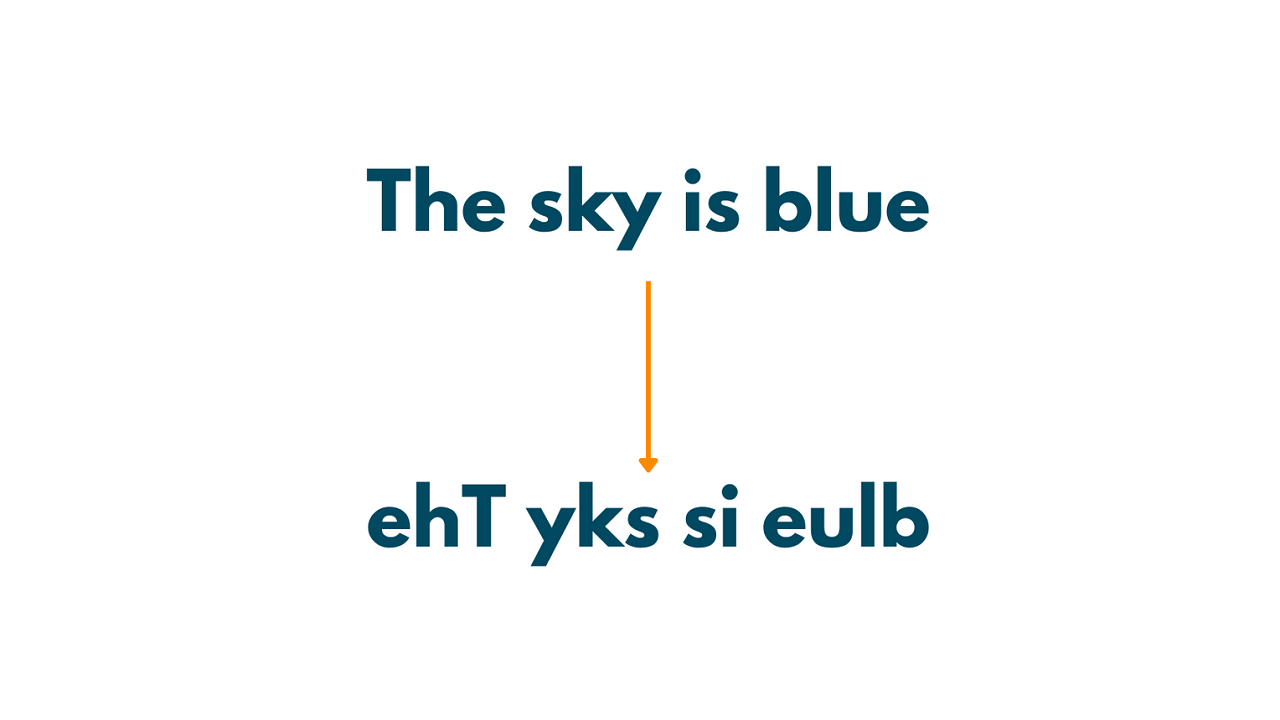# How to Reverse Words in a String? (using Loop & Recursion)

In this article, we'll explore some examples of how to reverse each word in a string using different approaches in C++. Each method has its own advantages and disadvantages, and the choice of method depends on the specific requirements of the problem at hand.

## How to Reverse Words in a String?

Reversing each word in a string is a common programming problem that involves reversing the order of the characters in each word while keeping the words themselves in the same order. This can be accomplished using a variety of different techniques, from built-in string functions to loops and recursion.

Here is an example:Let's learn about all the methods to do it one by one:

### Method 1) Using Built-In Functions

One of the simplest ways to reverse each word in a string is to use the built-in string functions in C++. Here's an example of how to do this:

```#include<iostream>
#include<string>
#include<algorithm>

using namespace std;

int main()
{
string sentence = "Hello World";
string reversed_sentence = "";

string word = "";
for (int i = 0; i < sentence.length(); i++) {
if (sentence[i] == ' ') {
reverse(word.begin(), word.end());
reversed_sentence += word + " ";
word = "";
}
else {
word += sentence[i];
}
}
reverse(word.begin(), word.end());
reversed_sentence += word;

cout << reversed_sentence << endl;

return 0;
}
```

Output:

```olleH dlroW
```

In this code, we first initialize an empty string called reversed_sentence, which we will use to store the final reversed sentence. We then loop through each character in the original sentence, building up a new string called word as we go.

When we encounter a space character, we reverse the order of the characters in the word using the reverse() function from the algorithm library, add the reversed word to the reversed_sentence string, and then reset the word string to empty. When we reach the end of the sentence, we reverse the final word and add it to the reversed_sentence string.

The Time Complexity of this method is  O(n), where n is the length of the string. This is because we are iterating over the string once to split it into words, and then again to concatenate the reversed words. The Space Complexity is O(n), where n is the length of the string.

### Method 2) Using a Loop

Another approach to reversing each word in a string is to use a loop to iterate over the words and then reverse each word individually. Here's an example of how to do this:

```#include<iostream>
#include<string>

using namespace std;

int main()
{
string sentence = "Hello World";

int start = 0, end = 0;
while (end <= sentence.length()) {
if (sentence[end] == ' ' || end == sentence.length()) {
for (int i = end - 1; i >= start; i--) {
cout << sentence[i];
}
cout << " ";
start = end + 1;
}
end++;
}

return 0;
}
```

Output:

`olleH dlroW`

In this code, we first iterate over each character in the string using a while loop. We find the end of each word by looking for a space character or the end of the string. Once we have identified the end of a word, we use a nested loop to iterate over each character in that word and print them out in reverse order. Finally, we add a space character after each word to separate them in the output.

The Time Complexity for this method is O(n), where n is the length of the string. This is because we are iterating over the string once to find the start and end indices of each word, and then again to reverse each word. The Space Complexity is O(1).

### Method 3) Using Recursion

In this method, we will use recursion to reverse each word in a string. The idea is to recursively reverse the substring of each word until we reach the end of the string.

Here is the Implementation in C++:

```#include<iostream>
#include<string>

using namespace std;

void reverseWord(string& str, int start, int end) {
if (start >= end) {
return;
}
swap(str[start], str[end]);
reverseWord(str, start + 1, end - 1);
}

string reverseWords(string& str) {
int start = 0, end = 0;
while (end <= str.length()) {
if (str[end] == ' ' || end == str.length()) {
reverseWord(str, start, end - 1);
start = end + 1;
}
end++;
}
return str;
}

int main() {
string sentence = "Hello World";
string reversedSentence = reverseWords(sentence);
cout << reversedSentence << endl;
return 0;
}```

Output:

`olleH dlroW`

In the `reverseWord` function, we pass the substring of a word, its start and end indices. If the start index is greater than or equal to the end index, we have reversed the entire word, so we simply return. Otherwise, we swap the characters at the start and end indices and recursively call the function on the remaining substring.

In the `reverseWords` function, we use a loop to find the start and end indices of each word in the string. We pass each word as a substring to the `reverseWord` function and reverse it recursively. Finally, we concatenate the reversed words to form the final output string.

The Time Complexity to reverse words in a string using recursion is O(n), where n is the length of the string. This is because we are iterating over the string once to find the start and end indices of each word, and then again recursively to reverse each word. The Space Complexity is O(n), where n is the length of the string.

## Conclusion

In this article, we have explored three different methods to reverse each word in a string using C++ programming language. While the first method uses built-in functions to split and join the string, the second and third methods use loops and recursion, respectively, to reverse each word.

### FavTutor - 24x7 Live Coding Help from Expert Tutors!